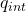Question

An isolated charged insulating solid sphere with radius of 20 cm is carrying charge of -4.8 x 10-16 A solid sphere is surrounded by a hollow charged to +4.8 x 10-16 C conducting sphere with inner radius of r; = 40 cm and outer radius ro = 50 cm Find an Electric field at

a) r= 30 cm
b) = 45 cm
c) = 60 cm Use following constants: K = 8.99 x 109 Nm2/C2, Ep = 8.854 x 10-12 C2/Nm2

1.thanhha

a)   E = -4.8 10⁻⁵ N / C
, b) E = 0
, c) E = 0

Explanation:

For this exercise let’s use Gauss’s law

Ф = E. dA =/ ε₀

As a Gaussian surface we use a sphere, whereby the electric field lines are parallel to the normal area, and the scalar product is reduced to the algebraic product

a) the field for r = 30 cm

At this point we place our Gaussian surface and see what charge there is inside, which is the charge of the solid sphere (r> 20cm), the charge on the outside does not contribute to the flow

E = q_{int} / A ε₀

q_{int} = -4.8 10-16 C

The area of ​​a sphere is

A = 4π R²

We replace

E = -4.8 10⁻¹⁶ / 4π 0.30² 8.85 10⁻¹²

E = -4.8 10⁻⁵ N / C

b) r = 45 cm

This point is inside the spherical conductor shell, as in an electric conductor in electrostatic equilibrium the charges are outside inside the shell there is no charge for which the field is zero

E = 0

c) R = 60 cm

This part is outside the two surfaces

The chare inside is

q_{int} = -4.8 10⁻¹⁶ + 4.8 10⁻¹⁶

q_{int} = 0

Therefore the electric field is

E = 0